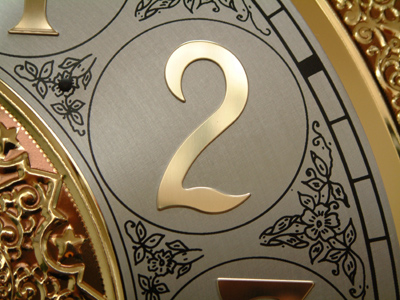What is 16 x 8 as a power of 2?

# Numbers - Powers

This Math quiz is called 'Numbers - Powers' and it has been written by teachers to help you if you are studying the subject at middle school. Playing educational quizzes is a fabulous way to learn if you are in the 6th, 7th or 8th grade - aged 11 to 14.

It costs only \$12.50 per month to play this quiz and over 3,500 others that help you with your school work. You can subscribe on the page at Join Us

Raising a number to a power is a POWERFUL operation, that's why I for Index comes before Division and Multiplication in the order of operations you know as BIDMAS. This quiz deals with the rules in Math for working with powers.

1.
What is 43 x 34 as a single power number?
127
712
77
none of these
You can't add the indices to multiply unless both numbers are powers of the same base
2.
What is 83 as a single power of 2?
26
27
28
29
83 = (2 x 2 x 2)3 = (23)3. To raise a power to a power, multiply the indices
3.
What is the value of 2-3?
1/3
1/6
1/8
1/9
A negative power is 1 divided by the positive power
4.
What is one hundred million as a power of 10?
105
106
107
108
The power of ten is the same as the number of zeroes. 100,000,000 has 8 noughts
5.
What is 54 ÷ 52?
5
25
125
625
To divide powers of the same base you subtract the indices; 5(4 - 2) = 52
6.
Which of these is not the same as 92 x 81?
94
38
89
6,561
9 x 9 x 9 x 9
7.
What is 16 x 8 as a power of 2?
25
26
27
28
16 = 24; 8 = 23. To multiply powers of the same number you add the indices
8.
What is 32 x 22 as a single power number?
64
62
54
none of these
3 x 3 x 2 x 2 = (3 x 2) x (3 x 2)
9.
Which of these gives the biggest number?
28
82
36
63
It's not always obvious which power number is the largest. The best way to check is to use a calculator!
10.
What is the value of 24?
8
16
32
64
24 (two to the power four) = 2 x 2 x 2 x 2
Author:  Frank Evans